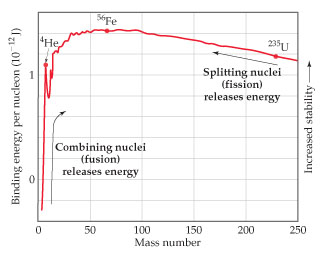# Problem: The atomic masses of hydrogen-2 (deuterium), helium-4, and lithium-6 are 2.014102 amu, 4.002602 amu, and 6.0151228 amu, respectively. For 6Li, calculate the nuclear binding energy in mega electronvolts (MeV) per nucleon.

🤓 Based on our data, we think this question is relevant for Professor Freschl's class at UWM.

###### FREE Expert Solution

We are asked to calculate the nuclear binding energy in mega electronvolts (MeV) per nucleon for  6Li which has an atomic mass of 6.0151228 amu

Nuclear binding energy is the minimum energy that would be required to disassemble the nucleus of an atom into its component parts. These component parts are neutrons and protons, which are collectively called nucleons

To calculate for the nuclear binding energy:

Step 1: Determine the number of protons and neutrons

Step 2: Determine the theoretical mass

Step 3: Calculate the mass defect (∆m)

Step 4: Determine nuclear binding energy per nucleon###### Problem Details

The atomic masses of hydrogen-2 (deuterium), helium-4, and lithium-6 are 2.014102 amu, 4.002602 amu, and 6.0151228 amu, respectively.For 6Li, calculate the nuclear binding energy in mega electronvolts (MeV) per nucleon.